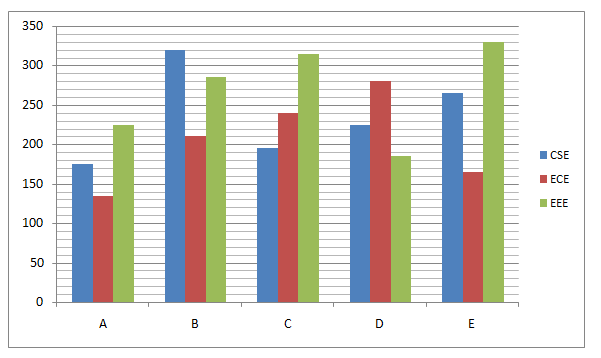# RRB PO Prelims Quantitative Aptitude (Day-06)

Dear Aspirants, Our IBPS Guide team is providing new series of Quantitative Aptitude Questions for RRB PO 2020 Prelims so the aspirants can practice it on a daily basis. These questions are framed by our skilled experts after understanding your needs thoroughly. Aspirants can practice these new series questions daily to familiarize with the exact exam pattern and make your preparation effective.

Start Quiz

Direction (1-5): Following question contains two equations as I and II. You have to solve both equations and determine the relationship between them and give answer as,

1)

I)2x2– 30x + 112 = 0

II) y2– 18y + 80 = 0

A) x > y

B) x ≥ y

C) x = y or relationship can’t be determined.

D) x < y

E) x ≤ y

2)

I)x2– 9x – 360 = 0

II) y2– 16y – 192 = 0

A) x > y

B) x ≥ y

C) x = y or relationship can’t be determined.

D) x < y

E) x ≤ y

3)

I) x2– 58x + 840 = 0

II) y2– 48y + 560 = 0

A) x > y

B) x ≥ y

C) x = y or relationship can’t be determined.

D) x < y

E) x ≤ y

4)

I) 3x2– 18x + 15 = 0

II) y2– 13y + 42 = 0

A) x > y

B) x ≥ y

C) x = y or relationship can’t be determined.

D) x < y

E) x ≤ y

5)

I) x2+ 39x + 224 = 0

II) y2+ 35y + 196 = 0

A) x > y

B) x ≥ y

C) x = y or relationship can’t be determined.

D) x < y

E) x ≤ y

Data Interpretation

Direction (6-10): Study the following information carefully and answer the questions given below.

The given bar graph shows the number of students in three different departments in five different colleges.6) The total number of ECE students in all the colleges together is approximately what percent of the number of CSE students in all the colleges together?

A) 87%

B) 89%

C) 85%

D) 83%

E) 91%

7) Total number of EEE students in all the colleges together is approximately what percent of the total number of students in E in all the departments together?

A) 173%

B) 176%

C) 179%

D) 182%

E) 185%

8) In which college the total number of students in all departments together is maximum?

A) C

B) D

C) E

D) A

E) B

9) What is the difference between the number of CSE students in B and the number of ECE students in C?

A) 60

B) 80

C) 100

D) 120

E) None of these

10) What is the ratio of the number of EEE students in D to the number of ECE students in A?

A) 17:7

B) 27:17

C) 37:27

D) 47:37

E) None of these

Directions (1-5) :

2x2 – 30x + 112 = 0

2x2 – 16x – 14x + 112 = 0

2x(x – 8) – 14(x – 8) = 0

(2x – 14)(x – 8) = 0

x = 8, 7

y2 – 18y + 80 = 0

y2 – 10y – 8y + 80 = 0

y(y – 10) – 8(y – 10) = 0

(y – 8)(y – 10) = 0

y = 8, 10

x ≤ y

x2 – 9x – 360 = 0

x2 – 24x + 15x – 360 = 0

x(x – 24) + 15(x – 24) = 0

(x + 15)(x – 24) = 0

x = – 15, 24

y2 – 16y – 192 = 0

y2 – 24y + 8y – 192 = 0

y(y – 24) + 8(y – 24) = 0

(y – 24)(y + 8) = 0

y = 24, -8

Relationship between x and y cannot be established.

x2 – 58x + 840 = 0

x2 – 30x – 28x + 840 = 0

x(x – 30) – 28(x – 30) = 0

(x – 28)(x – 30) = 0

x = 28, 30

y2 – 48y + 560 = 0

y2 – 20y – 28y + 560 = 0

y(y – 20) – 28(y – 20) = 0

(y – 28)(y – 20) = 0

y = 28, 20

x ≥ y

3x2 – 18x + 15 = 0

3x2 – 15x – 3x + 15 = 0

3x(x – 5) – 3(x – 5) = 0

(3x – 3)(x – 5) = 0

x = 1, 5

y2 – 13y + 42 = 0

y2 – 6y – 7y + 42 = 0

y(y – 6) – 7(y – 6) = 0

(y – 7)(y – 6) = 0

y = 7, 6

x < y

x2 + 39x + 224 = 0

x2 + 32x + 7x + 224 = 0

x(x + 32) + 7(x + 32) = 0

(x + 7)(x + 32) = 0

x = – 7, -32

y2 + 35y + 196 = 0

y2 + 7y + 28y + 196 = 0

y(y + 7) + 28(y + 7) = 0

(y + 28)(y + 7) = 0

y = -28, -7

Relationship between x and y cannot be established.

Directions (6-10) :

Required Percentage = [(135 + 210 + 240 + 280 + 165)/(175 + 320 + 195 + 225 + 265)] * 100

= 87%

Required percentage = [(225 + 285 + 315 + 185 + 330)/(265 + 165 + 330)] * 100

= 176%

A = 175 + 135 + 225 = 535

B = 320 + 210 + 285 = 815

C = 195 + 240 + 315 = 750

D = 225 + 280 + 185 = 690

E = 265 + 165 + 330 = 760

Difference = 320 – 240 = 80